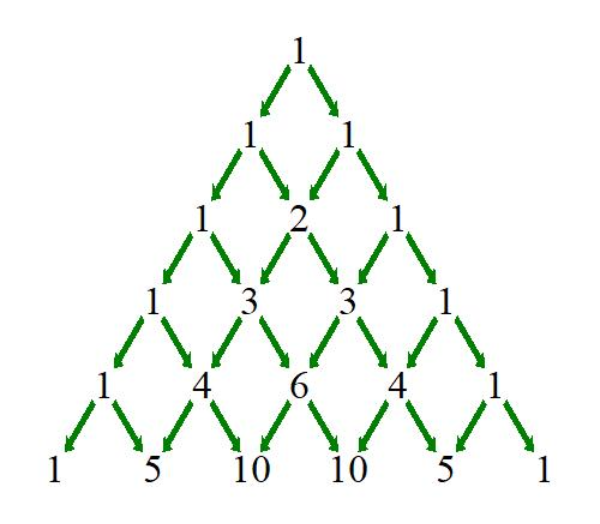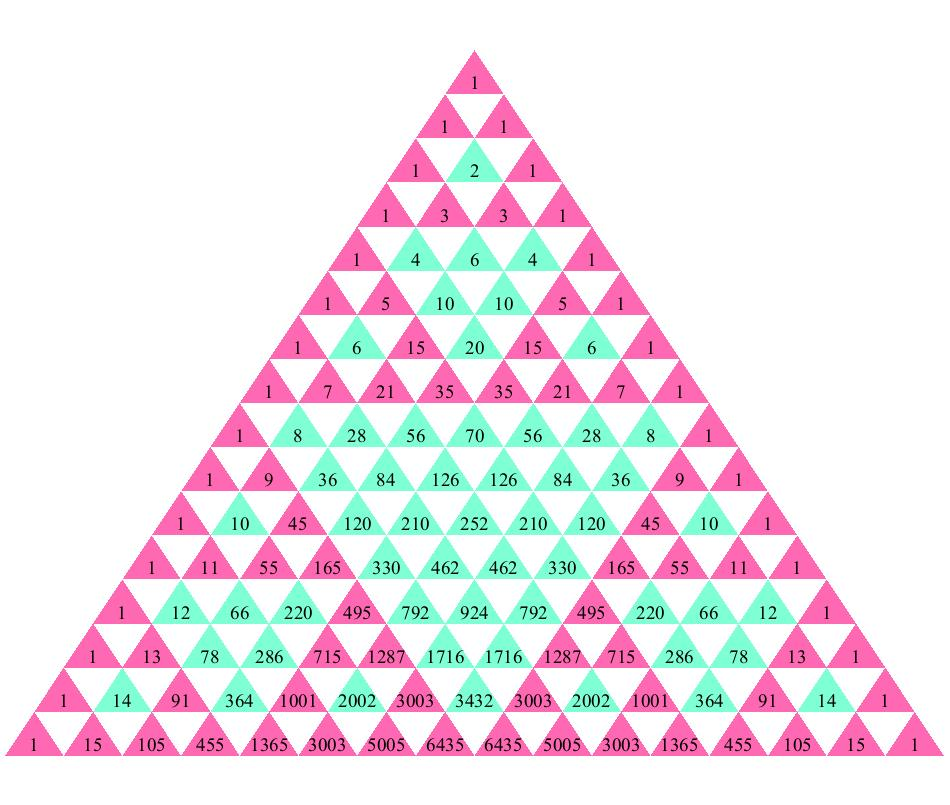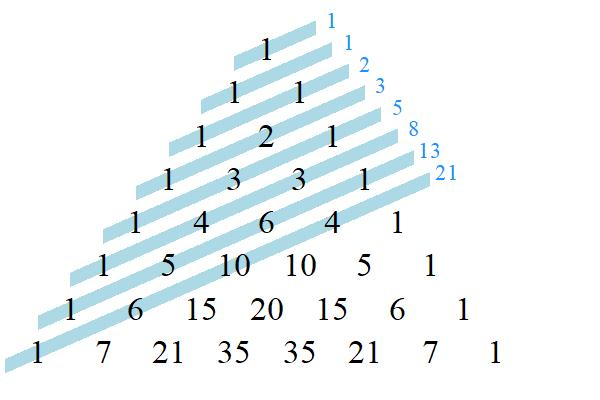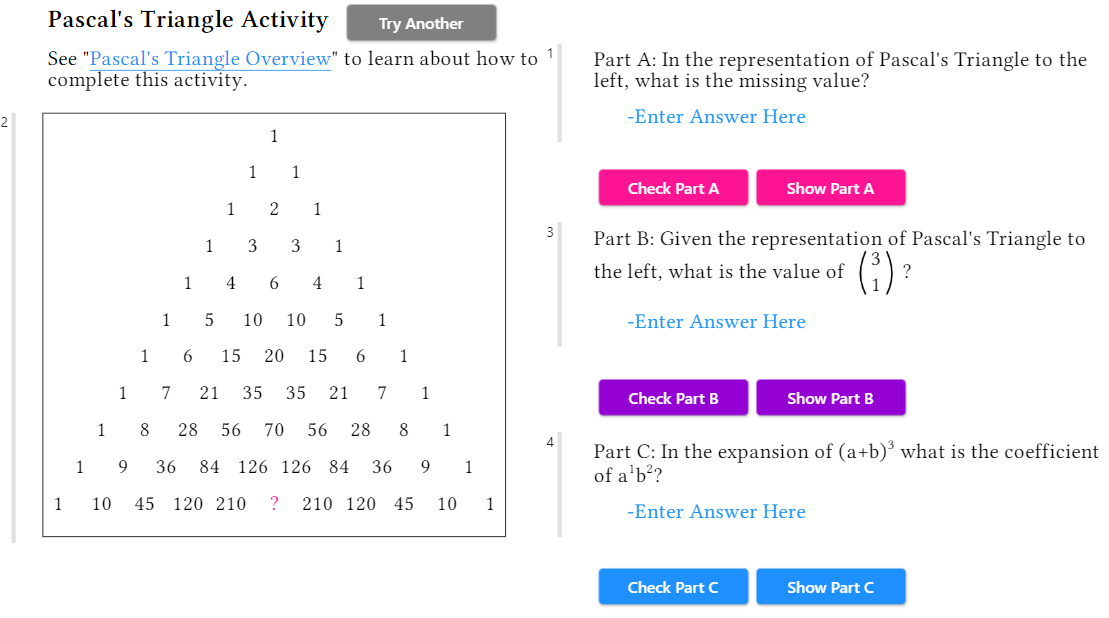## Pascal's Triangle Collection

Maple Learn

A new collection has been released on Maple Learn! The new Pascal’s Triangle Collection allows students of all levels to explore this simple, yet widely applicable array.Though the binomial coefficient triangle is often referred to as Pascal’s Triangle after the 17th-century mathematician Blaise Pascal, the first drawings of the triangle are much older. This makes assigning credit for the creation of the triangle to a single mathematician all but impossible.

Persian mathematicians like Al-Karaji were familiar with the triangular array as early as the 10th century. In the 11th century, Omar Khayyam studied the triangle and popularised its use throughout the Arab world, which is why it is known as “Khayyam’s Triangle” in the region. Meanwhile in China, mathematician Jia Xian drew the triangle to 9 rows, using rod numerals. Two centuries later, in the 13th century, Yang Hui introduced the triangle to greater Chinese society as “Yang Hui’s Triangle”. In Europe, various mathematicians published representations of the triangle between the 13th and 16th centuries, one of which being Niccolo Fontana Tartaglia, who propagated the triangle in Italy, where it is known as “Tartaglia’s Triangle”.

Blaise Pascal had no association with the triangle until years after his 1662 death, when his book, Treatise on Arithmetical Triangle, which compiled various results about the triangle, was published. In fact, the triangle was not named after Pascal until several decades later, when it was dubbed so by Pierre Remond de Montmort in 1703.

The Maple Learn collection provides opportunities for students to discover the construction, properties, and applications of Pascal’s Triangle. Furthermore, students can use the triangle to detect patterns and deduce identities like Pascal’s Rule and The Binomial Symmetry Rule. For example, did you know that colour-coding the even and odd numbers in Pascal’s Triangle reveals an approximation of Sierpinski’s Fractal Triangle?Or that taking the sum of the diagonals in Pascal's Triangle produces the Fibonacci Sequence?Learn more about these properties and discover others with the Pascal’s Triangle Collection on Maple Learn. Once you are confident in your knowledge of Pascal’s Triangle, test your skills with the interactive Pascal’s Triangle Activity﻿